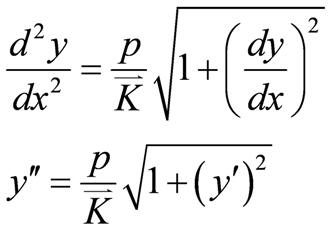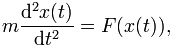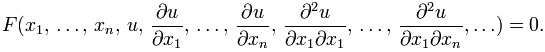#### Differential Equation Homework Help - K-12 Grade Level, College Level Mathematics

Introduction to Differential Equation

The differential equation is a mathematical equation for an unknown function of one or numerous variables which relates the values of the function itself and its derivatives of numerous orders. Differential equations play a very important role in physics, engineering, economics and many other disciplines.The differential equations occur in many regions of science and technology, specifically whenever a deterministic relation including some continuously changeable quantities and their rates of change in space and/or time (expressed as derivatives) is recognized or postulated. This is elaborated in traditional mechanics, where the motion of a body is defined by its position and velocity as the time value varies. The laws of Newton permit one (given the velocity, position, acceleration and numerous forces acting on the body) to convey these variables dynamically as a differential equation for the unknown position of the body as a function of time. Sometimes, this differential equation (known as equation of motion) might be solved explicitly. There are two main sections of Differential Equations that are described below:

Ordinary Differential Equation

In mathematics, an ordinary differential equation is an equation having a function of one independent variable and its derivatives. There are numerous common forms an ordinary differential equation can take, and these are categorized in practice as shown below. The derivatives are ordinary as partial derivatives only apply to functions of many independent variables.

A simple illustration for this is Newton's second law of motion - the relationship among the displacement x and the time t of the object under the force F that leads to the differential equation as follows:For the motion of a particle of constant mass m. Usually, F depends on the position x(t) of the particle at time t, and therefore the unknown function x(t) appears on both sides of the differential equation, as is displayed in the notation F(x(t)).

Partial Differential Equation

In mathematics, a partial differential equation is a differential equation which contains unknown multivariable functions and their partial derivatives. They are used to formulate the problems, related to the functions of numerous variables and are either answered by hand or used to form a relevant computer model.

A partial differential equation for the function u(x1,...xn) is as follows:The PDE is called linear, if F is a linear function of u and its derivatives. General examples of linear PDEs comprise equations like heat, wave, Laplace's, Helmholtz, Klein-Gordon and Poisson's equation.

Email based Differential Equation Homework Help -Assignment Help

Tutors at the www.tutorsglobe.com are committed to provide the best quality Differential equation homework help - assignment help. They use their experience, as they have solved thousands of the Differential equation assignments, which may help you to solve your difficult Differential equation homework. You can find solutions for all the topics come under the Differential equation. The dedicated tutors provide eminence work on your Math homework help and devoted to provide K-12 level Math to college level Math help before the deadline mentioned by the student. Differential equation homework help is available here for the students of school, college and university. TutorsGlobe assure for the best quality compliance to your homework. Compromise with quality is not in our dictionary. If we feel that we are not able to provide the homework help as per the deadline or given instruction by the student, we refund the money of the student without any delay.

Qualified and Experienced Differential equation Tutors at www.tutorsglobe.com

Tutors at the www.tutorsglobe.com take pledge to provide full satisfaction and assurance in Differential equation homework help. Students are getting Math homework help services across the globe with 100% satisfaction. We value all our service-users. We provide email based Differential equation homework help - assignment help. You can join us to ask queries 24x7 with live, experienced and qualified Math tutors specialized in Differential equation.